# Important Questions: Understanding Quadrilaterals Notes | Study Mathematics (Maths) Class 8 - Class 8

## Document Description: Important Questions: Understanding Quadrilaterals for Class 8 2022 is part of Understanding Quadrilaterals for Mathematics (Maths) Class 8 preparation. The notes and questions for Important Questions: Understanding Quadrilaterals have been prepared according to the Class 8 exam syllabus. Information about Important Questions: Understanding Quadrilaterals covers topics like Practice Exercise and Important Questions: Understanding Quadrilaterals Example, for Class 8 2022 Exam. Find important definitions, questions, notes, meanings, examples, exercises and tests below for Important Questions: Understanding Quadrilaterals.

Introduction of Important Questions: Understanding Quadrilaterals in English is available as part of our Mathematics (Maths) Class 8 for Class 8 & Important Questions: Understanding Quadrilaterals in Hindi for Mathematics (Maths) Class 8 course. Download more important topics related with Understanding Quadrilaterals, notes, lectures and mock test series for Class 8 Exam by signing up for free. Class 8: Important Questions: Understanding Quadrilaterals Notes | Study Mathematics (Maths) Class 8 - Class 8
 1 Crore+ students have signed up on EduRev. Have you?

Question 1: In the adjoining figure, find x + y + z + w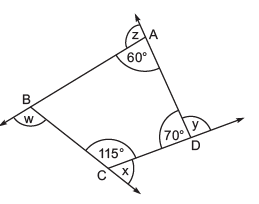Solution: Since, the sum of the measures of interior angles of a quadrilateral is 360°.
Also, 115° + 70° + 60° = 245°
∴ 245° +∠ABC = 360°
⇒∠ABC = 360° – 245° = 115°

Now, x = ext. ∠BCD
= 180° – ∠BCD
= 180° – 115° = 65°

Similarly, y = 180° – 70° = 110°
z = 180° – 60° = 120°
w = 180° – 115° = 65°
∴ x + y + z + w = 65° + 110° + 120° + 65°
= 360°

Question 2: In a quadrilateral ABCD, the angles A, B, C and D are in the ratio 1 : 2 : 3 : 4. Find the measure of each angle of the quadrilateral.
Solution: ∵∠A : ∠B : ∠C : ∠D = 1 : 2 : 3 : 4
∴ Let us suppose that

A = 1x°, B = 2x°

C = 3x°, –D = 4x°
Since, ∠A +∠B + ∠C +∠D = 360°
∴ x + 2x + 3x + 4x = 360°
⇒ 10x = 360°
⇒ x = 360/10 = 36°
∴ Angles are:
∠A = x° = 36°

∠B = 2x° = 2 x 36° = 72°
C = 3x° = 3 x 36° = 108°
D = 4x° = 4 x 36° = 144°

Thus, the measure of the angle of the quad. are 36°, 72°, 108° and 144°

Question 3: The interior angle of a regular is 108°. Find the number of sides of the polygon.
Solution: Let there are ‘n’ sides of the regular polygon.

Measure of each exterior angle =  (360/n)o
Since, the measure of each of the interior angle = 108°
Measure of each exterior angle = 180° – 108° = 72°
⇒ 360/n = 72   n = 360/72 = 5
Thus, the required number of sides of the regular polygon is 5.
Alternate Solution
Let there be ‘n’ sides of the regular polygon.
Since the measure of each interior angle is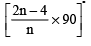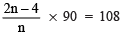⇒ (2n-4) x 90 = 108 x n

⇒ 180n - 360 = 108n

⇒ 180n - 108n = 360

⇒  72n = 360 ⇒ n= (360/72) = 5
Thus, there are 5 sides of the given regular polygon.

Question 4: Two regular polygons are such that the ratio of the measures their interior angles is 4 : 3 and the ratio between their number of sides is 2: 1. Find the number of sides of each polygon.
Solution: Let 2n and n be the number of sides of the regular polygons.
∴  Their interior angles are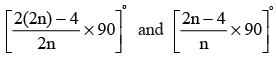Since the ratio of the interior angles is 4 : 3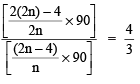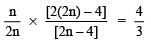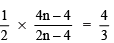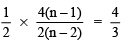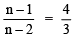⇒  3(n-1) = 4(n-2)

⇒  3n -3 = 4n -8

⇒  3n - 4n = -8 +3

⇒  -n = -5 ⇒ n=5

⇒   2n = 2 x 5 = 10
Thus, the number of sides of the polygons are 10 and 5 respectively.

Question 5: The exterior angle of a regular polygon is one-fifth of its interior angle. How many sides has the polygon?
Solution: Let the number of sides be ‘n’.
∴ Exterior angle of polygon =  (360/n)o

And the interior angle of the polygon =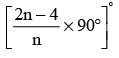since Exterior angle = 1/5 (Interior angle)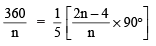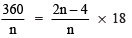⇒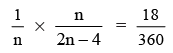⇒ 2n- 4 = 20 ⇒ 2n = 20 +4 = 24

⇒ n= 24/2 = 12

Thus, the polygon is having 12 sides.

Question 6: The measures of two adjacent angles of a parallelogram are in the ratio 4: 5. Find the measure of each of the angles of the parallelogram.
Solution: Let ABCD be a parallelogram such that ∠A and ∠B are 4x and 5x respectively.
Since, the adjacent angles are supplementary,

∴  ∠A+  ∠B = 1800

⇒  4x + 5x = 180o

⇒  9x+ = 1800   . ⇒   x = 180o/9 = 20o
∴ ∠A = 4x = 4 x 20° = 80°

and ∠B = 5x = 5 x 20° = 100°
We know that opposite angles of a parallelogram are equal.
∴ ∠C = ∠A = 80º

And  ∠D= ∠B = 100°
Thus,  ∠A = 80°, ∠B = 100°, ∠C = 80° and D = 100°

Question 7: In a quadrilateral ABCD, DO and CO are the bisectors of ∠D and ∠C respectively.
Prove that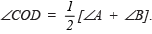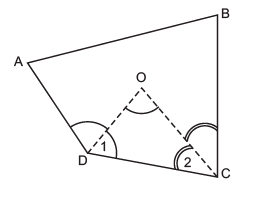Solution: In ΔCOD, we have

⇒ ∠COD + ∠1 + ∠2 = 180°
⇒ ∠COD = 180° – [–1 + –2]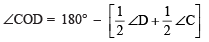⇒ But ∠A+ ∠B+∠C+∠D = 360o

⇒ ∠C+∠D = 360o - (∠A+∠B)

∴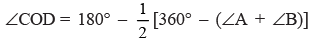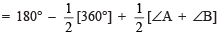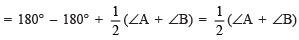Thus,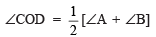Practice Exercise

Q 1. If an exterior angle of a regular polygon is 45°, then find the number of its sides.

Ans: 8

Q 2. If an interior angle of a regular polygon is 162°, then find the number of its sides.
Ans:
20

Q 3. Find x in the following figure.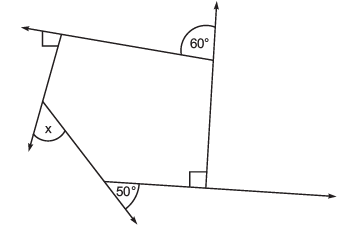Ans:
70o

Q 4. Find the measure of an interior angle of a regular polygon having 15 sides.

Ans: 156o

Q 5. Find the measure of each of the (i) exterior angle. (ii) interior angle of an octagon.

Ans: (i) 45° (ii) 135°

Q 6. An angle of a parallelogram measures 70°. Find the measure of the remaining three angles.

Ans: 110°, 70°, 110°

Q 7. In the following figure, ABCD is a parallelogram. Find measures of x, y and z.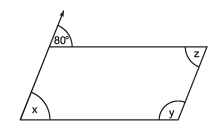Ans: x = 80°, y = 100°, z = 80°

Q 8. In the following figure, find the measures of x, y, z and w.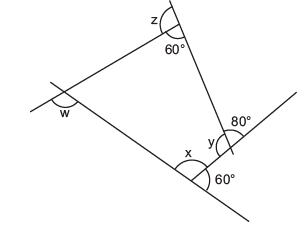Ans: x = 120°, y = 100°, z = 120°, w = 80°

Q 9. One angle of a quadrilateral is 111° and the remaining three angles are equal. Find three angles.

Ans: 81° each

Q.10. What is the ratio of the interior angles of a pentagon and a decagon?

Note: (i) A pentagon has 5 sides.  (ii) A decagon has 10 sides.

Ans: 3: 4

The document Important Questions: Understanding Quadrilaterals Notes | Study Mathematics (Maths) Class 8 - Class 8 is a part of the Class 8 Course Mathematics (Maths) Class 8.
All you need of Class 8 at this link: Class 8

## Mathematics (Maths) Class 8

187 videos|321 docs|48 tests
 Use Code STAYHOME200 and get INR 200 additional OFF

## Mathematics (Maths) Class 8

187 videos|321 docs|48 tests

Track your progress, build streaks, highlight & save important lessons and more!

,

,

,

,

,

,

,

,

,

,

,

,

,

,

,

,

,

,

,

,

,

;